Publicité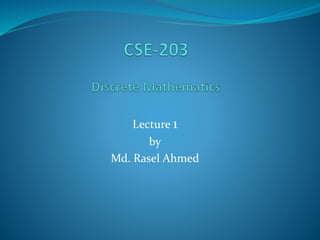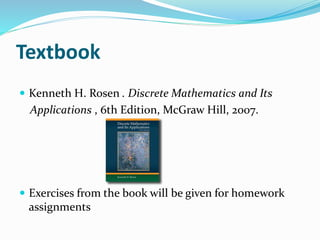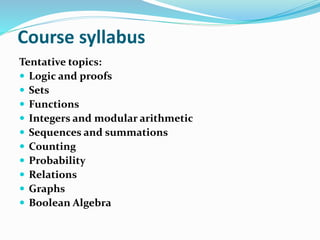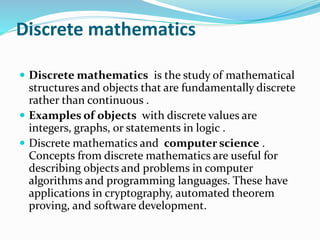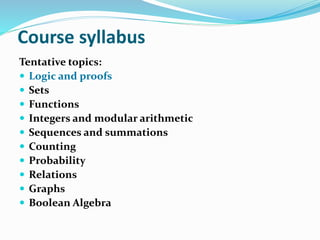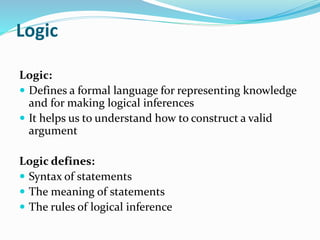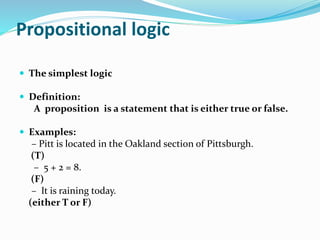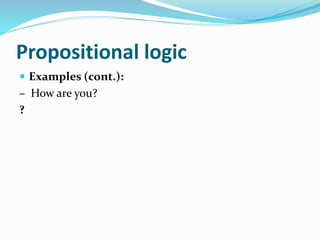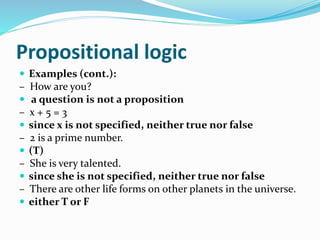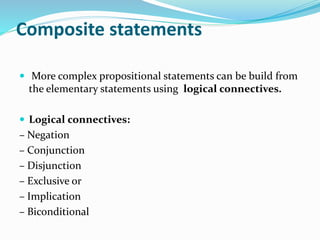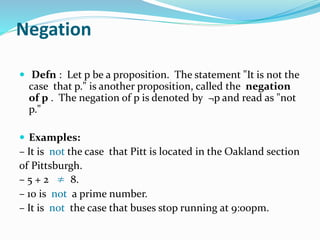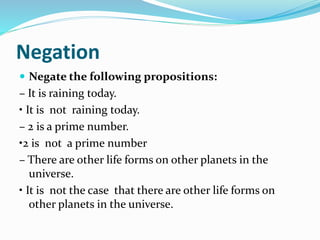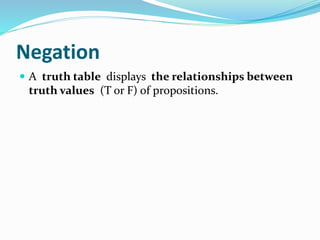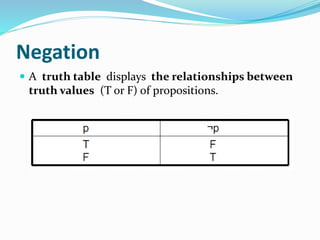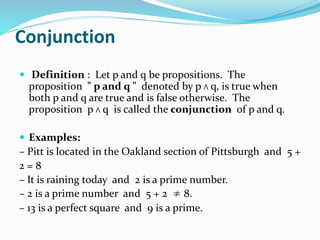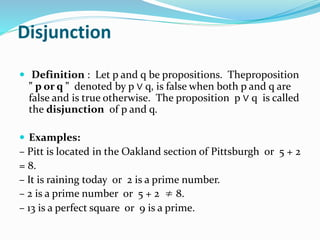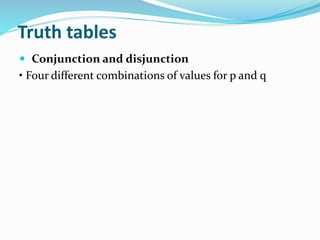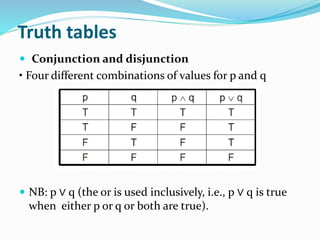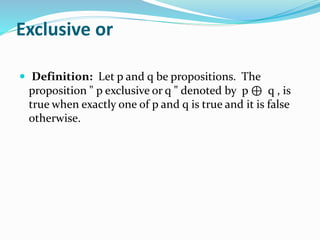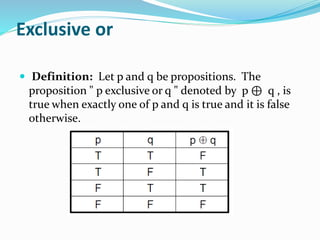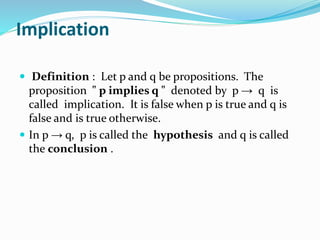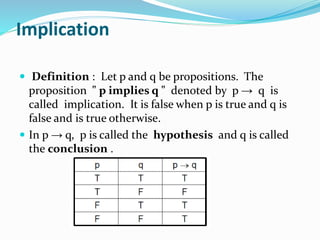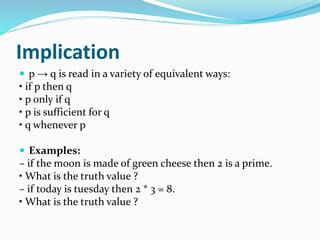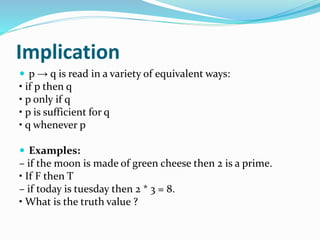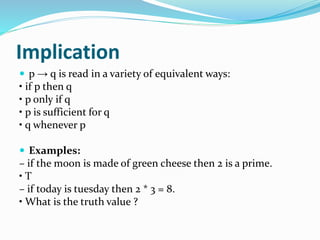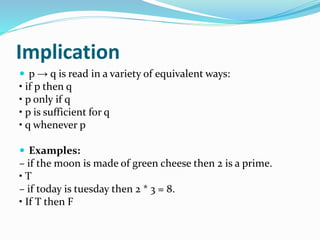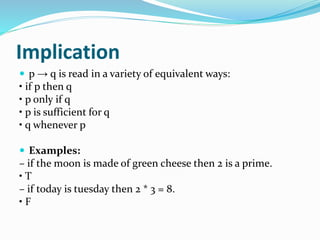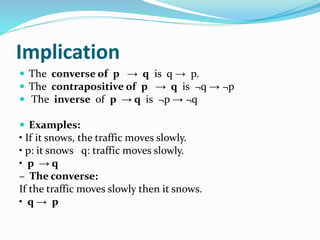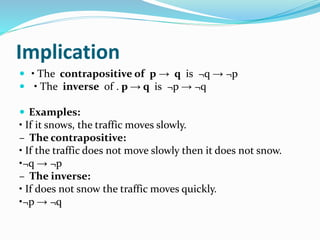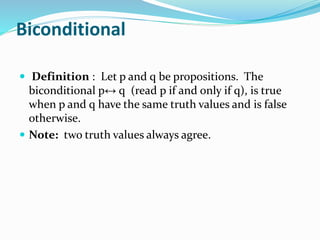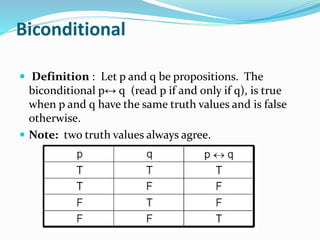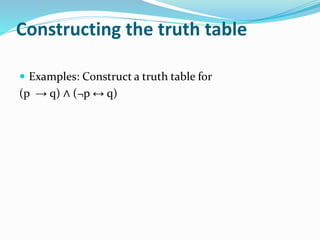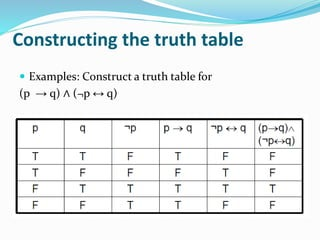1 sur 36
Publicité

### CSE-203 Lec_1.pptx

1. Lecture 1 by Md. Rasel Ahmed
2. Instructor: Md. Rasel Ahmed Lecturer Dept. Of CSE Faridpur Engineering College Room No # 207 E-mail: raseljucse@gmail.com Lecture hours: Tuesday: 11.20 a.m. - 12.10 p.m. Wednesday: 11.20 a.m. - 12.10 p.m. Thursday: 9.40 a.m. - 10.30 a.m.
3. Textbook  Kenneth H. Rosen . Discrete Mathematics and Its Applications , 6th Edition, McGraw Hill, 2007.  Exercises from the book will be given for homework assignments
4. Course syllabus Tentative topics:  Logic and proofs  Sets  Functions  Integers and modular arithmetic  Sequences and summations  Counting  Probability  Relations  Graphs  Boolean Algebra
5. Discrete mathematics  Discrete mathematics is the study of mathematical structures and objects that are fundamentally discrete rather than continuous .  Examples of objects with discrete values are integers, graphs, or statements in logic .  Discrete mathematics and computer science . Concepts from discrete mathematics are useful for describing objects and problems in computer algorithms and programming languages. These have applications in cryptography, automated theorem proving, and software development.
6. Course syllabus Tentative topics:  Logic and proofs  Sets  Functions  Integers and modular arithmetic  Sequences and summations  Counting  Probability  Relations  Graphs  Boolean Algebra
7. Logic Logic:  Defines a formal language for representing knowledge and for making logical inferences  It helps us to understand how to construct a valid argument Logic defines:  Syntax of statements  The meaning of statements  The rules of logical inference
8. Propositional logic  The simplest logic  Definition: A proposition is a statement that is either true or false.  Examples: – Pitt is located in the Oakland section of Pittsburgh. (T) – 5 + 2 = 8. (F) – It is raining today. (either T or F)
9. Propositional logic  Examples (cont.): – How are you? ?
10. Propositional logic  Examples (cont.): – How are you?  a question is not a proposition – x + 5 = 3  since x is not specified, neither true nor false – 2 is a prime number.  (T) – She is very talented.  since she is not specified, neither true nor false – There are other life forms on other planets in the universe.  either T or F
11. Composite statements  More complex propositional statements can be build from the elementary statements using logical connectives.  Logical connectives: – Negation – Conjunction – Disjunction – Exclusive or – Implication – Biconditional
12. Negation  Defn : Let p be a proposition. The statement "It is not the case that p." is another proposition, called the negation of p . The negation of p is denoted by ¬p and read as "not p."  Examples: – It is not the case that Pitt is located in the Oakland section of Pittsburgh. – 5 + 2 ≠ 8. – 10 is not a prime number. – It is not the case that buses stop running at 9:00pm.
13. Negation  Negate the following propositions: – It is raining today. • It is not raining today. – 2 is a prime number. •2 is not a prime number – There are other life forms on other planets in the universe. • It is not the case that there are other life forms on other planets in the universe.
14. Negation  A truth table displays the relationships between truth values (T or F) of propositions.
15. Negation  A truth table displays the relationships between truth values (T or F) of propositions.
16. Conjunction  Definition : Let p and q be propositions. The proposition " p and q " denoted by p Λ q, is true when both p and q are true and is false otherwise. The proposition p Λ q is called the conjunction of p and q.  Examples: – Pitt is located in the Oakland section of Pittsburgh and 5 + 2 = 8 – It is raining today and 2 is a prime number. – 2 is a prime number and 5 + 2 ≠ 8. – 13 is a perfect square and 9 is a prime.
17. Disjunction  Definition : Let p and q be propositions. Theproposition " p or q " denoted by p ∨ q, is false when both p and q are false and is true otherwise. The proposition p ∨ q is called the disjunction of p and q.  Examples: – Pitt is located in the Oakland section of Pittsburgh or 5 + 2 = 8. – It is raining today or 2 is a prime number. – 2 is a prime number or 5 + 2 ≠ 8. – 13 is a perfect square or 9 is a prime.
18. Truth tables  Conjunction and disjunction • Four different combinations of values for p and q
19. Truth tables  Conjunction and disjunction • Four different combinations of values for p and q  NB: p ∨ q (the or is used inclusively, i.e., p ∨ q is true when either p or q or both are true).
20. Exclusive or  Definition: Let p and q be propositions. The proposition " p exclusive or q " denoted by p ⊕ q , is true when exactly one of p and q is true and it is false otherwise.
21. Exclusive or  Definition: Let p and q be propositions. The proposition " p exclusive or q " denoted by p ⊕ q , is true when exactly one of p and q is true and it is false otherwise.
22. Implication  Definition : Let p and q be propositions. The proposition " p implies q " denoted by p → q is called implication. It is false when p is true and q is false and is true otherwise.  In p → q, p is called the hypothesis and q is called the conclusion .
23. Implication  Definition : Let p and q be propositions. The proposition " p implies q " denoted by p → q is called implication. It is false when p is true and q is false and is true otherwise.  In p → q, p is called the hypothesis and q is called the conclusion .
24. Implication  p → q is read in a variety of equivalent ways: • if p then q • p only if q • p is sufficient for q • q whenever p  Examples: – if the moon is made of green cheese then 2 is a prime. • What is the truth value ? – if today is tuesday then 2 * 3 = 8. • What is the truth value ?
25. Implication  p → q is read in a variety of equivalent ways: • if p then q • p only if q • p is sufficient for q • q whenever p  Examples: – if the moon is made of green cheese then 2 is a prime. • If F then T – if today is tuesday then 2 * 3 = 8. • What is the truth value ?
26. Implication  p → q is read in a variety of equivalent ways: • if p then q • p only if q • p is sufficient for q • q whenever p  Examples: – if the moon is made of green cheese then 2 is a prime. • T – if today is tuesday then 2 * 3 = 8. • What is the truth value ?
27. Implication  p → q is read in a variety of equivalent ways: • if p then q • p only if q • p is sufficient for q • q whenever p  Examples: – if the moon is made of green cheese then 2 is a prime. • T – if today is tuesday then 2 * 3 = 8. • If T then F
28. Implication  p → q is read in a variety of equivalent ways: • if p then q • p only if q • p is sufficient for q • q whenever p  Examples: – if the moon is made of green cheese then 2 is a prime. • T – if today is tuesday then 2 * 3 = 8. • F
29. Implication  The converse of p → q is q → p.  The contrapositive of p → q is ¬q → ¬p  The inverse of p → q is ¬p → ¬q  Examples: • If it snows, the traffic moves slowly. • p: it snows q: traffic moves slowly. • p → q – The converse: If the traffic moves slowly then it snows. • q → p
30. Implication  • The contrapositive of p → q is ¬q → ¬p  • The inverse of . p → q is ¬p → ¬q  Examples: • If it snows, the traffic moves slowly. – The contrapositive: • If the traffic does not move slowly then it does not snow. •¬q → ¬p – The inverse: • If does not snow the traffic moves quickly. •¬p → ¬q
31. Biconditional  Definition : Let p and q be propositions. The biconditional p↔ q (read p if and only if q), is true when p and q have the same truth values and is false otherwise.  Note: two truth values always agree.
32. Biconditional  Definition : Let p and q be propositions. The biconditional p↔ q (read p if and only if q), is true when p and q have the same truth values and is false otherwise.  Note: two truth values always agree.
33. Constructing the truth table  Examples: Construct a truth table for (p → q) ∧ (¬p ↔ q)
34. Constructing the truth table  Examples: Construct a truth table for (p → q) ∧ (¬p ↔ q)
Publicité